# Engineering Mechanics - Moments of Inertia

## Why Engineering Mechanics Moments of Inertia?

In this section you can learn and practice Engineering Mechanics Questions based on "Moments of Inertia" and improve your skills in order to face the interview, competitive examination and various entrance test (CAT, GATE, GRE, MAT, Bank Exam, Railway Exam etc.) with full confidence.

## Where can I get Engineering Mechanics Moments of Inertia questions and answers with explanation?

IndiaBIX provides you lots of fully solved Engineering Mechanics (Moments of Inertia) questions and answers with Explanation. Solved examples with detailed answer description, explanation are given and it would be easy to understand. All students, freshers can download Engineering Mechanics Moments of Inertia quiz questions with answers as PDF files and eBooks.

## Where can I get Engineering Mechanics Moments of Inertia Interview Questions and Answers (objective type, multiple choice)?

Here you can find objective type Engineering Mechanics Moments of Inertia questions and answers for interview and entrance examination. Multiple choice and true or false type questions are also provided.

## How to solve Engineering Mechanics Moments of Inertia problems?

You can easily solve all kind of Engineering Mechanics questions based on Moments of Inertia by practicing the objective type exercises given below, also get shortcut methods to solve Engineering Mechanics Moments of Inertia problems.

### Exercise :: Moments of Inertia - General Questions

• Moments of Inertia - General Questions
1.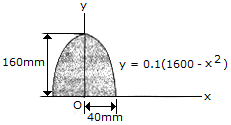Determine the radius of gyration ky of the parabolic area.

 A. ky = 76.5 mm B. ky = 17.89 mm C. ky = 78.6 mm D. ky = 28.3 mm

Explanation:

No answer description available for this question. Let us discuss.

2.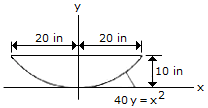Determine the inertia of the parabolic area about the x axis.

 A. Ix = 11,430 in.4 B. Ix = 32,800 in.4 C. Ix = 13,330 in.4 D. Ix = 21,300 in.4

Explanation:

No answer description available for this question. Let us discuss.

3.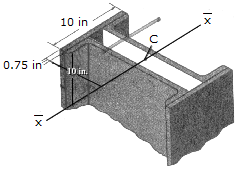The composite cross section for the column consists of two cover plates riveted to two channels. Determine the radius of gyration k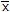with respect to the centroidalaxis. Each channel has a cross-sectional area of Ac = 11.8 in.2 and moment of inertia (I)c = 349 in.4.

 A. k = 7.74 in. B. k = 6.29 in. C. k = 5.44 in. D. k = 4.25 in.

Explanation:

No answer description available for this question. Let us discuss.

4.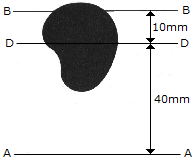The irregular area has a moment of inertia about the AA axis of 35 (106) mm4. If the total area is 12.0(103) mm2, determine the moment of inertia if the area about the BB axis. The DD axis passes through the centroid C of the area.

 A. IBB = 5.00(106 ) mm4 B. IBB = 17.00(106 ) mm4 C. IBB = 16.80(106 ) mm4 D. IBB = 55.4(106 ) mm4